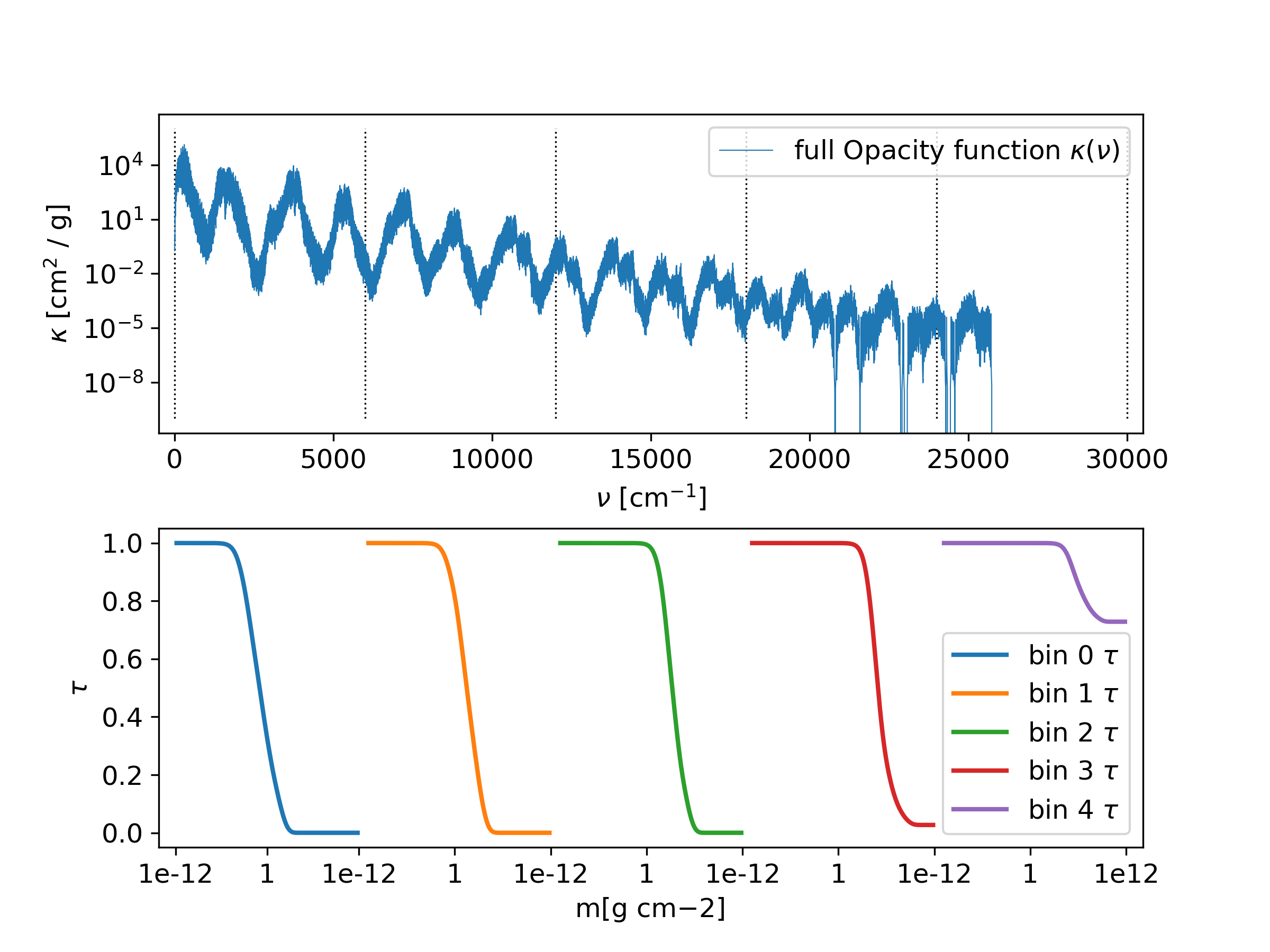# The transmission function¶

With the doTransmission option in the param.dat file, the transmissions function $$\tau$$

$\tau = \int_0^\infty e^{-\kappa \tilde{m}} d\nu = \int_0^1 e^{-\kappa \tilde{m}} dy$

can be calculated for a set of column masses $$\tilde{m}$$, where $$\tilde{m}$$ is set according to:

$\tilde{m}_i = e^{((i - nTr/2) \cdot dTr)}.$

The parameters nTr and dTr can be set in the param.dat file. The transmission function is stored in the file Out<name>_tr.dat,

An example of the transmission function for fife bins is shown in Fig. 10.

Relevant parameters for this example:
• doStoreFullK = 1

• doTransmission = 2

• nbins = 5

• nTr = 1000

• dTr = 0.05Fig. 10 Transmission function